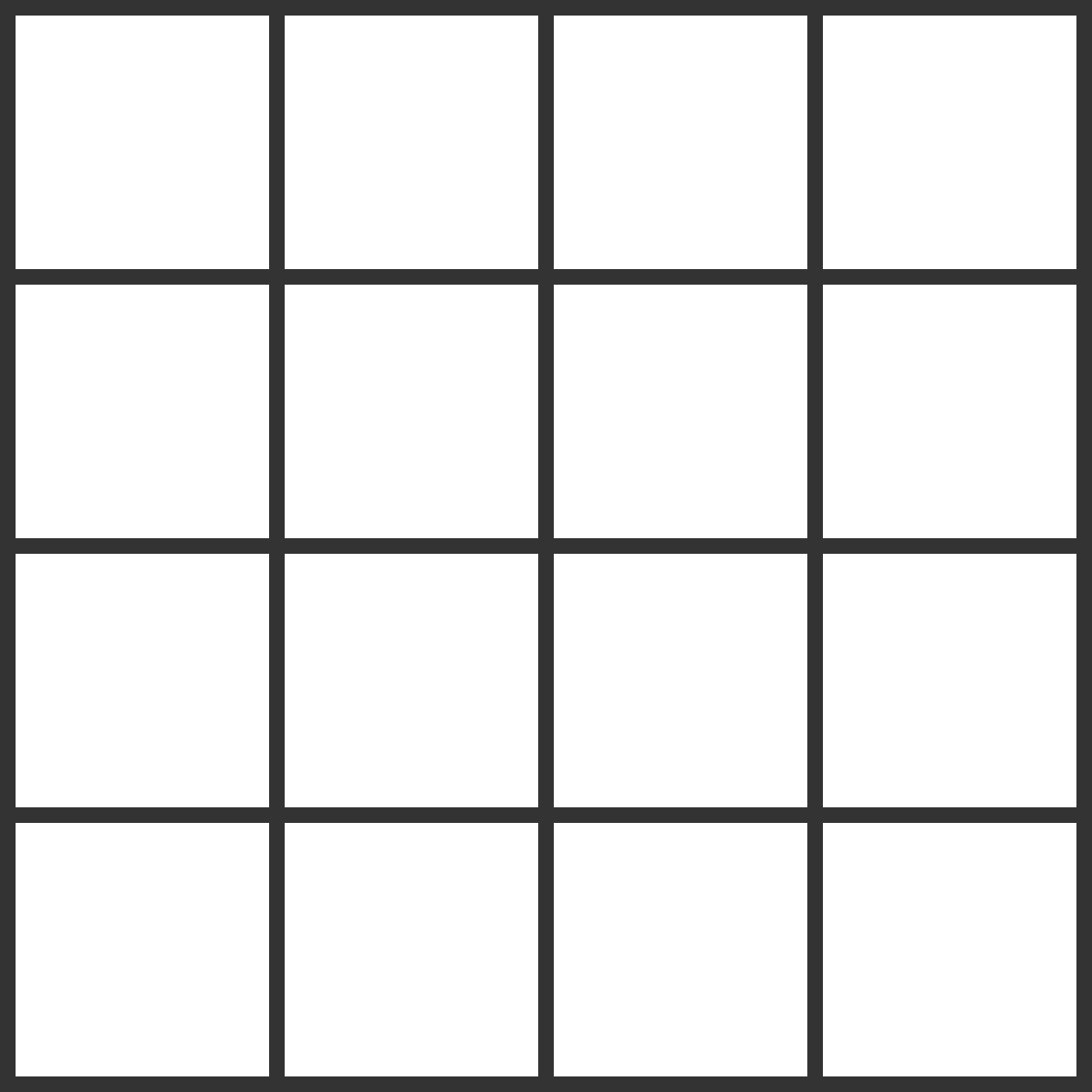# 4x4

Below is a $4\times 4$ grid. I want to fill in each cell with exactly one positive integer such that

• all 16 numbers are distinct,
• each number is less than or equal to 30, and
• the product of the 4 numbers in any row, column, or diagonal is equal.

Is this possible?×